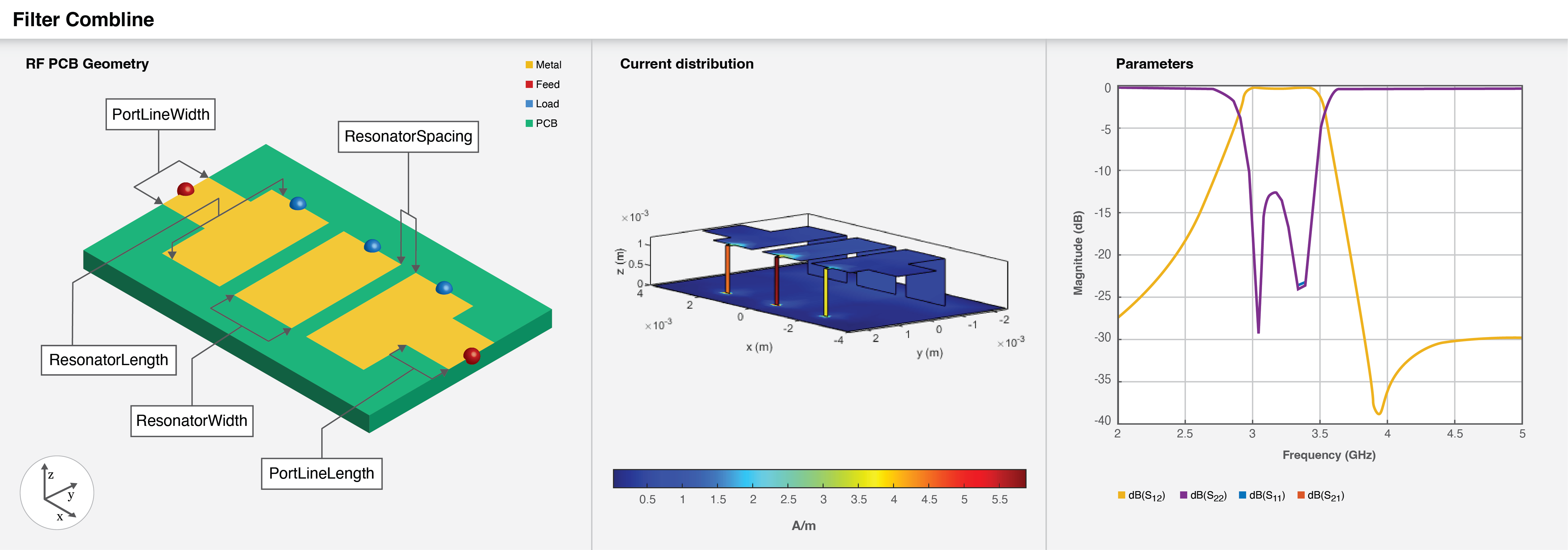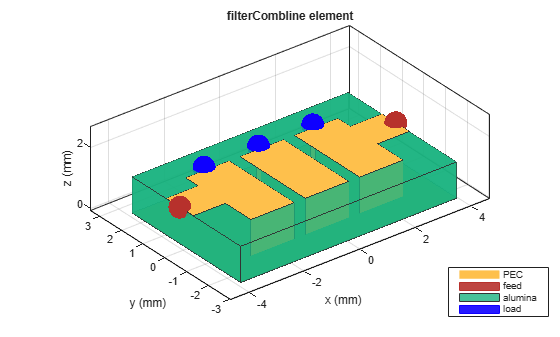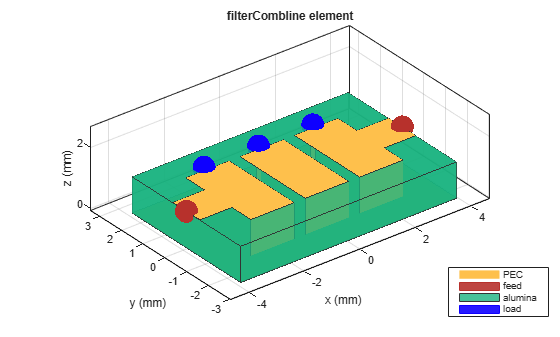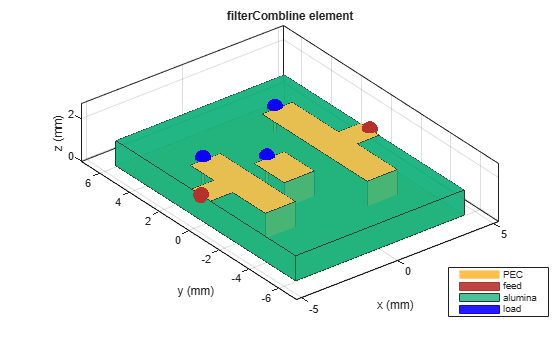# filterCombline

Create combline filter in microstrip form

Since R2022b

## Description

Use the `filterCombline` object to create a combline filter in the microstrip form.## Creation

### Syntax

``filter = filterCombline``
``filter = filterCombline(Name=Value)``

### Description

example

````filter = filterCombline` creates a default combline line filter using an Alumina substrate with the passband of the filter centered around 3.1 GHz.```

example

````filter = filterCombline(Name=Value)` sets Properties using one or more name-value arguments. For example, `filterCombline(ResonatorWidth=0.0016)` creates a combline filter with a resonator width of `0.0016` meters. Properties not specified retain their original values.```

## Properties

expand all

Order of the filter, specified as a positive scalar.

Example: `filter = filterCombline(FilterOrder=4)`

Data Types: `double`

Length of the resonator in meters, specified as a positive scalar or a vector of positive elements equal in size to `FilterOrder`.

Example: ```filter = filterCombline(ResonatorLength=0.007)```

Data Types: `double`

Width of the resonator in meters, specified as a positive scalar.

Example: ```filter = filterCombline(ResonatorWidth=0.0017)```

Data Types: `double`

Spacing between the resonators in meters, specified as a positive scalar or a vector of positive elements equal in size to (`FilterOrder`-1).

Example: ```filter = filterCombline(ResonatorSpacing=0.0008)```

Data Types: `double`

Y-offset for each resonator in meters, specified as a positive scalar or a vector of positive elements equal in size to `FilterOrder`. If the value is a scalar, all the resonators have the same offset along the Y-axis.

Example: ```filter = filterCombline(ResonatorOffset=(0 0.1 0.2))```

Data Types: `double`

Length of the input and output lines in meters, specified as a positive scalar.

Example: ```filter = filterCombline(PortLineLength=0.0014)```

Data Types: `double`

Width of the input and output lines in meters, specified as a positive scalar.

Example: ```filter = filterCombline(PortLineWidth=0.0087)```

Data Types: `double`

Y-offset for the input and output lines in meters, specified as a positive scalar.

Example: `filter = filterCombline(FeedOffset=0.0087)`

Data Types: `double`

Capacitor value in farad, specified as a positive scalar.

Example: `filter = filterCombline(Capacitor=2e-12)`

Data Types: `double`

Height of the combline filter from the ground plane in meters, specified as a positive scalar. For multilayer dielectrics, use the `Height` property to create the filter between the two dielectric layers.

Example: `filter = filterCombline(Height=0.0028)`

Data Types: `double`

Width of the ground plane in meters, specified as a positive scalar.

Example: ```filter = filterCombline(GroundPlaneWidth=0.0048)```

Data Types: `double`

Type of dielectric material used as a substrate, specified as a `dielectric` object. The dielectric material in a `filterCombline` object with default properties is Alumina.

Example: `d = dielectric("FR4");` `filter = filterCombline(Substrate=d)`

Type of metal used in the conducting layers, specified as a `metal` object. The type of metal in a `filterCombline` object with default properties is PEC.

Example: `m = metal("Copper");` `filter = filterCombline(Conductor=m)`

## Object Functions

 `charge` Calculate and plot charge distribution `current` Calculate and plot current distribution `design` Design coupled line filter around specified frequency `feedCurrent` Calculate current at feed port `getZ0` Calculate characteristic impedance of transmission line `layout` Plot all metal layers and board shape `mesh` Change and view mesh properties of metal or dielectric in PCB component `shapes` Extract all metal layer shapes of PCB component `show` Display PCB component structure or PCB shape `sparameters` Calculate S-parameters for RF PCB objects

## Examples

collapse all

Create a default combline filter.

`filter = filterCombline`
```filter = filterCombline with properties: FilterOrder: 3 ResonatorLength: 0.0030 ResonatorWidth: 0.0016 ResonatorSpacing: 4.0000e-04 ResonatorOffset: 0 PortLineLength: 0.0011 PortLineWidth: 0.0013 FeedOffset: 3.0000e-04 Capacitor: 1.0000e-12 Height: 0.0012 GroundPlaneWidth: 0.0050 Substrate: [1x1 dielectric] Conductor: [1x1 metal] ```

View the filter.

`show(filter)`Create a combline filter with the feed offset equal to zero.

`filter = filterCombline('FeedOffset',0);`

Visualize the filter.

`show(filter);`Create a third-order combline filter with a different resonator offset.

```filter = filterCombline(FilterOrder=3,ResonatorLength=[0.005,0.002,0.007],... ResonatorSpacing=[0.6e-3,1.2e-3],ResonatorOffset=[0.001e-3,0,0.5e-3], ... GroundPlaneWidth=12e-3);```

Visualize the filter.

`show(filter);`Pozar, David M. Microwave Engineering. 4th ed. Hoboken, NJ: Wiley, 2012.

 Al-Yasir, Y., R.A. Abd-Alhameed, J.M. Noras, A.M. Abdulkhaleq, and N.O. Parchin. “Design of Very Compact Combline Band-Pass Filter for 5G Applications.” In Loughborough Antennas & Propagation Conference 2018 (LAPC 2018), 61 (4 pp.)-61 (4 pp.). Loughborough, UK: Institution of Engineering and Technology, 2018

 Hong, Jia-Sheng. "Microstrip Filters for RF/Microwave Applications". 2nd ed. Wiley Series in Microwave and Optical Engineering. Hoboken, N.J: Wiley, 2011.